## 1. Overview

In this tutorial, we’ll talk about the latent space in deep learning. First, we’ll define the term and discuss its importance to deep learning, and then we’ll present some examples of latent space.

## 2. Definition

Formally, a latent space is defined as an abstract multi-dimensional space that encodes a meaningful internal representation of externally observed events. Samples that are similar in the external world are positioned close to each other in the latent space.

To better understand the concept, let’s think about how humans perceive the world. We are able to understand a broad range of topics by encoding each observed event in a compressed representation in our brain.

For example, we don’t keep in mind every detail of the appearance of a dog to be able to recognize a dog in the street. As we can see in the illustration below, we keep an internal representation of the general appearance of a dog: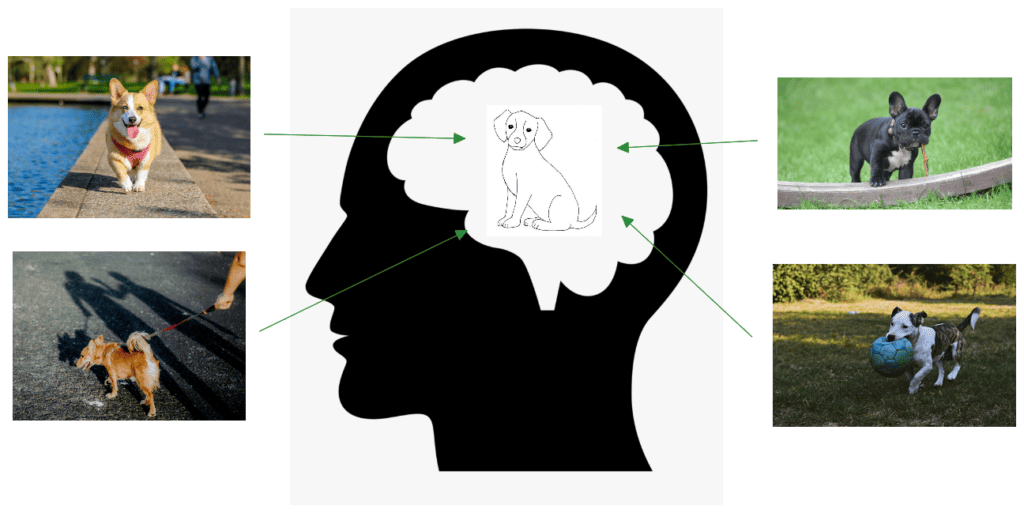In a similar way, the latent space tries to provide a compressed understanding of the world to a computer through a spatial representation.

## 3. Importance in Deep Learning

Deep learning has revolutionized many aspects of our life with applications ranging from self-driving cars to predicting serious diseases. Its main goal is to transform the raw data (such as the pixel values of an image) into a suitable internal representation or feature vector from which the learning subsystem, often a classifier, could detect or classify patterns in the input. So, we realize that deep learning and latent space are strongly related concepts since the internal representations of the former constitute the latter.

As we can see below, a deep learning model takes as input raw data and outputs discriminative features that lie in a low-dimensional space referred to as latent space. These features are then used to solve various tasks like classification, regression, or reconstruction: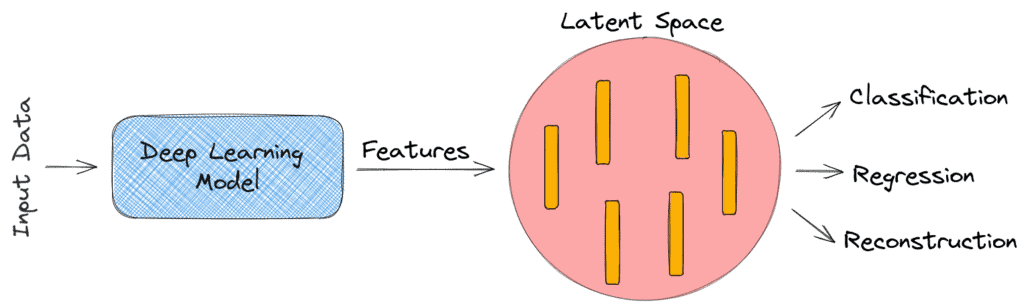To better understand the importance of latent space in deep learning, we should think of the following question: Why do we have to encode the raw data in a low-dimensional latent space before classification, regression, or reconstruction?

The answer is data compression. Specifically, in cases where our input data are high-dimensional, it is impossible to learn important information directly from the raw data.

For example, in an image classification task, the input dimensions could bethat correspond toinput pixels. It seems impossible for a system to learn useful patterns for classification by looking at so many values. The solution is to encode the high-dimensional input space to a low-dimensional latent space using a deep neural network.

## 4. Examples

Now, let’s discuss some examples in deep learning where the existence of a latent space is necessary to capture the task complexity and achieve high performance.

### 4.1. Image Feature Space

As we mentioned earlier, latent space is an indispensable part of every convolutional neural network that takes as input the raw pixels of an image and encodes in the final layer some high-level features that lie in a latent space.

This latent space enables the model to perform the task (e.g., classification) using low-dimensional discriminative features instead of the high-dimensional raw pixels. In the image below, we can see the general architecture of a CNN: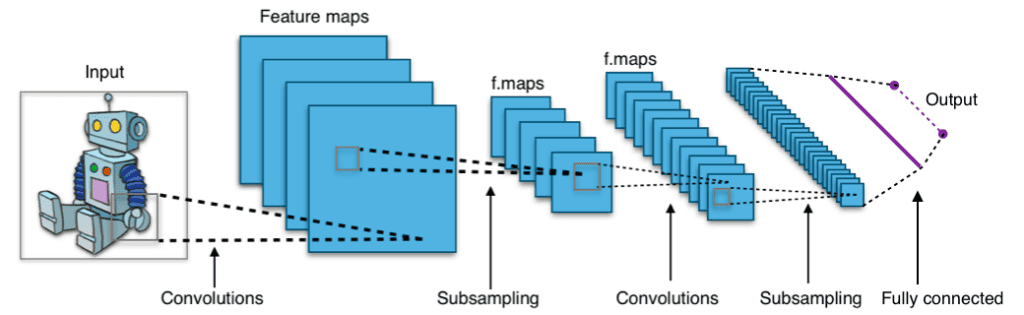After training, the last layer of the model has captured the important patterns of the input that are needed for the image classification task. In the latent space, images that depict the same object have very close representations. Generally, the distance of the vectors in the latent space corresponds to the semantic similarity of the raw images.

Below, we can see how the latent space of an animal classification model may seem. The green points correspond to the latent vector of each image extracted from the last layer of the model. We observe that vectors of the same animals are closer to the latent space. Therefore, it is easier for the model to classify the input images using these feature vectors instead of the raw pixel values: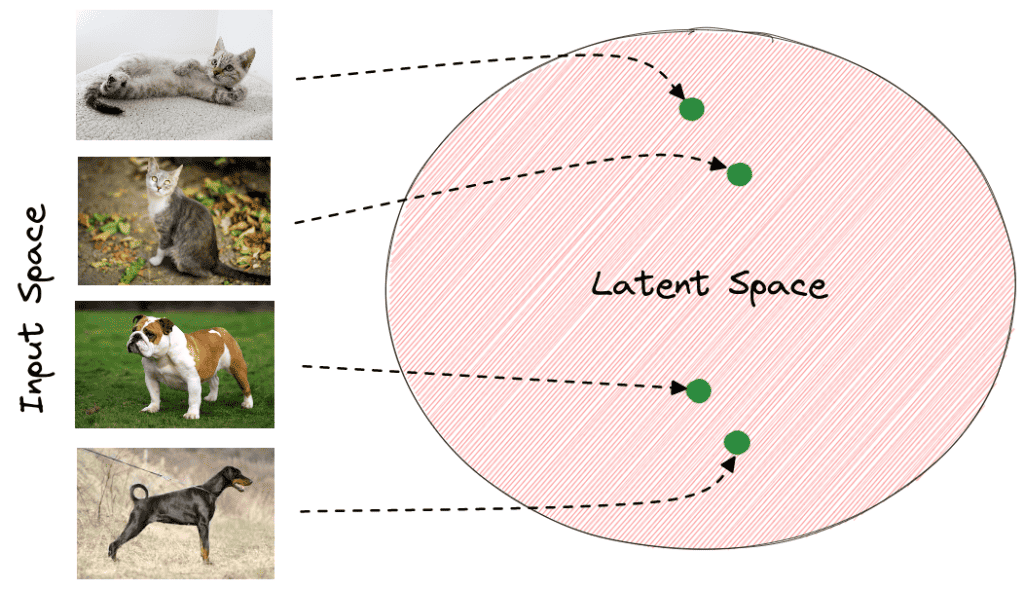### 4.2. Word Embedding Space

In natural language processing, word embeddings are numerical representations of words so that similar words have close representations. So, word embeddings lie in a latent space where every word is encoded into a low-dimensional semantic vector.

There are many algorithms for learning word embeddings like Word2Vec or GloVe. In the image below, we can see an illustration of the topology of the word embeddings in the latent space: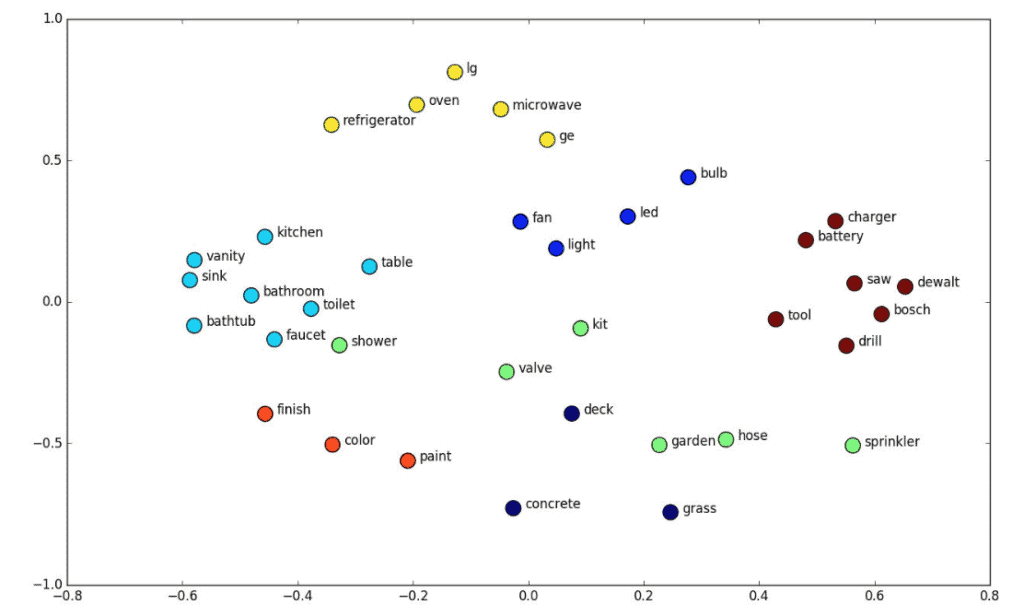As expected, semantically similar words like the word ‘toilet’ and the word ‘bathroom’ have close word embeddings in the latent space.

### 4.3. GANs

In previous tutorials, we have talked a lot about GANs and their applications. Briefly, a GAN takes as input a random vectorfrom some prior distribution and outputs and image. The goal of the model is to learn to generate the underlying distribution of the real dataset. For example, if our dataset contains images with chairs, the GAN model learns to generate synthetic images with chairs.

The input of a GAN acts as a latent vector since it encodes the output imagein a low-dimensional vector. To verify this, we can see how interpolation works in the latent space since we can handle specific attributes of the image by linearly modifying the latent vector.

In the image below, we can see how we can handle the pose of a face by changing the latent vector of the GAN that generates it:## 5. Conclusion

In this tutorial, we made an introduction to the latent space in deep learning. First, we discussed the definition of the term and its strong relation with deep learning, and then we presented some illustrative examples.